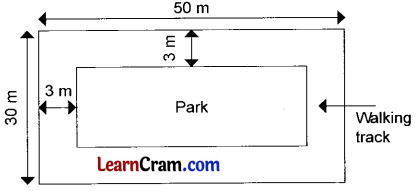# DAV Class 6 Maths Chapter 15 Worksheet 2 Solutions

The DAV Class 6 Maths Book Solutions and DAV Class 6 Maths Chapter 15 Worksheet 2 Solutions of Perimeter and Area offer comprehensive answers to textbook questions.

## DAV Class 6 Maths Ch 15 WS 2 Solutions

Question 1.
The area of a plastic sheet is 300 sq cm and its length is 30 cm. What is the breadth of the plastic sheet?
Area of the plastic sheet = 300 sq. cm
Its length = 30 cm
Breadth of the plastic sheet = $$\frac{\text { Area }}{\text { Length }}$$
= $$\frac{300}{30}$$
= 10 cm
Hence the required breadth = 10 cm.

Question 2.
The area of a football ground is 2900 m2. If its breadth is 29 m, find the length of the ground.
The area of the football ground
= 2900 m2
Length of the ground = $$\frac{\text { Area }}{\text { Breadth }}$$
= $$\frac{2900}{29}$$
= 100 m
Hence the required length = 100 m.

Question 3.
The perimeter of a square lawn is 72 m. Find the length of the side of the square lawn.
Perimeter of the square lawn = 72 m
Side = $$\frac{1}{4}$$ × Perimeter
= $$\frac{1}{4}$$ × 72 m
= 18 m
Hence the required length of side = 18 m.Question 4.
A rectangular garden is 230 m long and its area is 13800 m2. Find the width of the garden.
Area of the rectangular garden = 13800 m2
Its length = 230 m
The width of the garden = $$\frac{\text { Area }}{\text { Length }}$$
= $$\frac{13800}{230}$$
= 60 m
Hence the required width = 60 m.

Question 5.
Neha covered 95.52 m by running around a square garden. Find the length of each side of the garden.
Perimeter of a square garden = 95.52 m
Side = $$\frac{\text { Perimeter }}{4}$$
= $$\frac{95.52}{4}$$
= 23.88 m
Hence the side of the square garden – 23.88 m

### DAV Class 6 Maths Chapter 15 Value Based Questions

Question 1.
On the occasion of Republic Day, a school organised a poster making competition for the students of Class VI. The topic was ‘National Integration’. Each child was given a square sheet of side 60 cm. They had to make a border of width 2 cm using the coloured tape and the remaining portion had to be divided into four equal portions to showT main teachings of different religions.
(a) Find the length of the coloured tape needed for the border.Side of square sheet = 60 cm
The coloured taped needed for the border = Perimeter of the square
= 4 × side
= 4 × 60
= 240 cm

(b) What will be the side of the square into which the sheet has been divided?
The side of the square (ABCD) into which the sheet has been divided
= $$\frac{60-2 \times 2}{2}=\frac{60-4}{2}=\frac{56}{2}$$ = 28 cm

(c) What do you learn from the given situation?
To inculcate team work among children.Question 2.
There was a deserted land near Rajesh’s house. The colony people use to throw garbage on that land. Rajesh along with his friends contacted the people of the colony and they all decided to develop the land into a park with a walking track where the children can play and elders can walk.(a) Find the cost for levelling the park including the track at the rate of ₹ 15 per square metre.